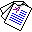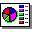# Coordination between Logical Agents

## Chiaki Sakama and Katsumi Inoue

Proceedings of the 5th International Workshop on Computational Logic in Multi-Agent Systems (CLIMA-V), Lecture Notes in Artificial Intelligence 3487, pages 161-177, 2005.

## Abstract

In this paper we suppose an agent that has a knowledge base written in logic programming and sets of beliefs under the answer set semantics. We then consider the following two problems: given two logic programs P1 and P2, which have the sets of answer sets AS(P1) and AS(P2), respectively; (i) find a program Q which has the set of answer sets such that AS(Q)=AS(P1) ∪ AS(P2); (ii) find a program R which has the set of answer sets such that AS(R)=AS(P1) ∩ AS(P2). A program Q satisfying the condition (i) is called generous coordination of P1 and P2; and R satisfying (ii) is called rigorous coordination of P1 and P2. Generous coordination retains all of the original belief sets of each agent, but admits the introduction of additional belief sets of the other agent. By contrast, rigorous coordination forces each agent to give up some belief sets, but the result remains within the original belief sets for each agent. We provide methods for constructing these two types of coordination and discuss their properties.

Keywords: answer set programming, coordination, program composition.Full Paper (pdf 166K)Slide (pdf 94K) © Springer-Verlag